# Definition

Here we will introduce the most important notion related to sequences: the limit of a sequence. Before giving a formal definition we will try to get some feeling for what is a limit.

Recall the example of the geometric series {(1/2)n}n = 0,1,2,....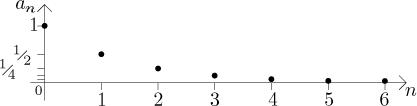If we calculate the first several terms and express them in the decimal form, we get

{1, 0.5, 0.25, 0.125, 0.0625, 0.03125, 0.015625, 0.0078125, 0.00390625, 0.001953125, 0.0009765625,...}

This partial list as well as the picture suggest the same: as we progress along the sequence, its terms are getting closer and closer to 0, in fact we can get them as close to zero as we want just by looking far enough. We would say that 0 is the limit of this sequence; we can also say that this sequence converges to 0. Generally speaking, the problem of convergence and of a limit is really an attempt to answer the question "what happens with the expression an when n gets really really large". In this example, the answer would be: "When n is really very large, then (1/2)n is about 0."

We used the above example to get some basic idea of what a limit is. However, it is not the best example, since it may suggest some things about limits that are not true. We will now address the more popular misconceptions by trying to specify more precisely what a limit is.

A number L is a limit of a given sequence {an} if

1. the numbers an eventually get as close to the number L as you would want; that is, the distance |an − L| eventually gets smaller than any given tolerance, and
2. the distance |an − L| not only eventually gets as close to zero as wanted, but it also stays that close.

In order to understand these two requirements we look at two examples of sequences for which the indicated L is not a limit: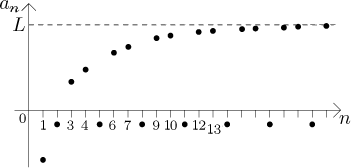In this example, the sequence satisfies the condition (1). Indeed, the numbers an get closer to the level L, and from the picture it would seem that when somebody gives us a tolerance, however small, we can find some an that are within this tolerance from L.
However, this sequence does not satisfy the condition (2), because every third term jumps down below the n-axis, which is far from L, and the picture suggests that this is happening again and again throughout the sequence. Therefore, although we can find some an arbitrarily close to L, we cannot make the sequence stay that close as we go to the right.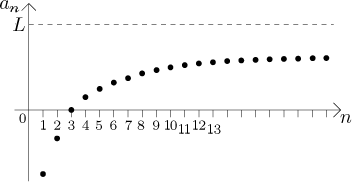The sequence in this picture satisfies in some sense (2), that is, it keeps getting closer and closer to L and stays that way, but the condition (1) does not seem to be true; the picture suggests that the sequence is leveling off too early to reach the level L (although of course it would be dangerous to make any definite conclusions from just a picture; perhaps the sequence does reach the level L, it just goes there really really slowly; we do not really know).

The last example is also interesting for another reason. In many books, when they want to express in words what a limit is, they would say: Limit is a number to which the given sequence gets closer and closer. Actually, I sometimes say it, too, because it is short and does give some idea. However, it might be misleading, since in the last picture, the terms of the sequence are getting closer and closer to L, yet it is not a limit (it seems). The "arbitrary closedness" bit is really crucial.

There is a way to express both conditions simultaneously. It goes like this:

When somebody gives us a tolerance (traditionally denoted by ε, the Greek letter epsilon), however small, we should be able to disregard some beginning part of this sequence so that that rest of it, the "tail", already stays within this tolerance from L.

In the first non-convergent picture we can see that if somebody gives us tolerance ε = L, that is, exactly the distance between the level L and the n-axis, then no matter how large a beginning of the sequence we disregard, there will be always the points in the tail that jump below the n-axis and therefore they are not within the given tolerance from L.

In the second picture, if we choose for ε say half of the distance between the level L and the level at which the sequence seems to settle down,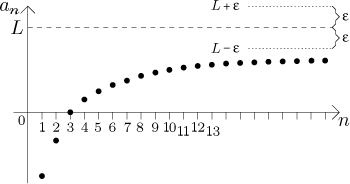then obviously no matter how much of the sequence we cut off, the remainder will never stay within this tolerance; in fact, it will never even satisfy it with a single term (at least it looks that way).

So now we have some idea of what the limit means. It is equally important to understand conditions that are not required for a limit. To dispell the most frequent misconception, we emphasise this:

When a sequence converges to some number L, it is not necessary for its terms to get closer and closer to the level L in some special way.

For instance, in the very first example the sequence {(1/2)n}n=0,1,2,... had limit 0 and its points were getting to this level all from the top and gradually, the sequence was decreasing. This is a very special example and it is in fact too nice to give the proper idea of a limit. For that we turn to the next example: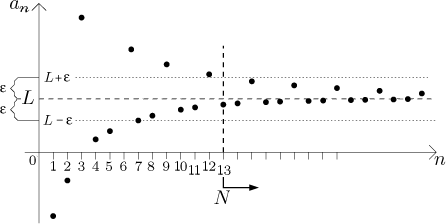We note several things. The sequence is actually a combination of two trends. Similarly to the first picture of a sequence where L was not a limit, also here every third term "jumps away", this time not below the n-axis, but above the level L and quite far from it. The terms that are left after disregarding those jumping terms form a nice sequence that seems to approach the level L from below in a nice gentle curve. We would guess that they by themselves form a sequence that converges to L. But what about the whole given sequence? Note that although every third term jumps away, this time the jumps are progressively closer and closer to the level L. We claim that the whole given sequence in the picture converges to L. Indeed, the condition (1) above is satisfied and this time also (2) works.

We can try to see it from the point of view of the description with epsilon. When somebody gives us a tolerance ε as in the picture, we check how much of the "nice part" we have to disregard so that the rest of it stays within the tolerance and we also check how many of the "jumping terms" we have to disregard. Eventually we realize that we have to cut away the beginning of the sequence as indicated by the thick vertical line and the rest of the sequence is already within the tolerance. The index where we cut off the tail is important, traditionally it is denoted by N. In our picture we have N = 12 and we see that the truncated sequence {a12, a13, a14, a15,...} stays within the tolerance.

Now we are ready to give the formal definition.

Definition.
Consider a sequence {an}. We say that a real number L is a limit of this sequence as n tends to infinity, or that the sequence converges to this L as n tends to infinity, if for every ε > 0 there is some natural number N so that for all n = N, N + 1, N + 2,... we have |an − L| < ε.
The notation is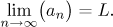We can also write "anL as n→∞".

If a sequence has a limit, the sequence is called convergent, or we say that it converges. Otherwise it is called divergent, or we say that it diverges.

Note that from the notation we do not see at what index the sequence starts. This is not needed: the limit of a sequence (if there is any) does not depend on the first terms of the given sequence, only on terms "near the end". Also, when talking about a limit, we usually skip the "as n tends to infinity" part, since when talking about a sequence, n cannot really go any other way. For more details on notation see this note.

The best way to understand this definition is to think of it in terms of a game. Somebody - your opponent - chooses the tolerance ε, typically he would choose it very small to make your life more difficult. Your task is to guarantee that the sequence will stay within this tolerance by cutting away its beginning. That is, you have to find the cutoff index N so that when you look at aN and also all the following terms of the sequence, their distance from L is less than the given tolerance.

In the last example we actually suggested a proof by definition that the given sequence converges to L. However, we did only one step of it. The key to the definition is that you have to be able to win the game no matter how small the given epsilon is, in other words, for all possible positive epsilons. From the picture it is clear that if somebody gives you a smaller epsilon than the one in the picture, you would have to move the cutoff point further to the right but you could still find it.
Compare this to the second picture of a sequence not converging to L above. There, if epsilon is quite large, we can win the game. But if somebody comes up with a small epsilon, the game cannot be won.Convergent sequences are "nice". Their main feature is that we can replace all an for n large with a number L without making a large error. In fact, given the maximal error we can make, we know that it is enough to disregard some terms at the beginning of the sequence and the rest can be replaced by L without exceeding this error.

Example: We claim that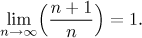The sequence goes (in decimal form) {2, 1.5, 1.333..., 1.250, 1.200, 1.166..., 1.1428...,...}, so the claim does not seem suspicious. The picture is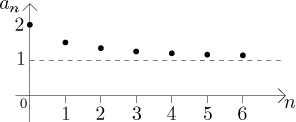For the formal proof by definition that the limit is correct see here.

There is an alternative way of visualizing sequences and limits which will come handy later, when talking about functions. You can find it in this note.

When investigating convergence, it is important to understand what can make a sequence divergent. There are two root causes:

Oscillation whose size does not decrease to zero. Recall this example:The terms of this sequence oscillate (for large n) between levels of approximately L and approximately -L/5. Thus the size of oscillation stays large. We argued that L indicated in the picture is not a limit of this sequence. Similar argument can be made that no number L' can be a limit, since if we choose L' different from L, then the points of the sequence that are getting closer and closer to L will stay away from this new L'. In the picture we indicate ε for which the game from the definition cannot be won when you attempt to prove that L' is the limit.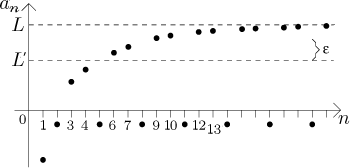Unchecked growth. Recall the example of the geometric sequence {2n}n=0,1,2,....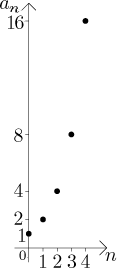We know from Theory - Introduction - Important examples that this sequence is not bounded from above. Now if you imagine a horizontal line on some level L in the picture, it would seem clear that the sequence "runs away" from it, so the chosen number L cannot be a limit. For a more precise argument look here.

In this situation people would often say that the sequence "blows up". It can also blow up in the direction down, when the numbers are larger and larger in absolute value, but with negative sign. A sequence may also blow up in both directions, then it would also necessarily have large oscillations as some points are getting large and positive and some "very negative".

Any sequence that does not converge incorporates one or both of these behaviours. There is one kind of divergent sequence where we can still give some useful information. Namely, in the last example, the terms of the sequence go steadily up without any bound. While the "steadily" part is not necessary, the tendency of "going up and eventually staying there" is very useful and deserves a name.

Definition.
Conside a sequence {an}. We say that infinity is a limit of this sequence as n tends to infinity, or that the sequence tends to infinity as n tends to infinity, if for every real number K there is some natural number N so that for all n = N, N + 1, N + 2,... we have an > K.
The notation is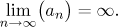We can also write "an→∞ as n→∞".

We say that negative infinity is a limit of this sequence as n tends to infinity, or that the sequence tends to negative infinity as n tends to infinity, if for every real number K there is some natural number N so that for all n = N, N + 1, N + 2,... we have an < K.
The notation is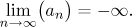We can also write "an→−∞ as n→∞".

If a sequence has a limit infinity or negative infinity, we say that the limit exists.

Here the game works as follows. For the infinite limit, somebody picks a level K, typically a very large number. To win you have to find a cutoff point N so that the tail aN, aN+1, aN+2,... of the sequence stays above this level K.

For the negative infinity, somebody picks a level K, typically a very large number with a negative sign (a number "far low" in a picture). To win you have to find a cutoff point N so that the tail aN, aN+1, aN+2,... of the sequence stays below this level K.

We will now look at some examples: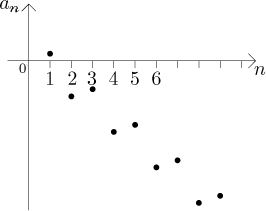In this picture, the sequence is not monotone, it actually oscillates in a seemingly regular pattern, but as it goes, it eventually drops below any level you care to choose and stays there. So by definition, it tends to negative infinity.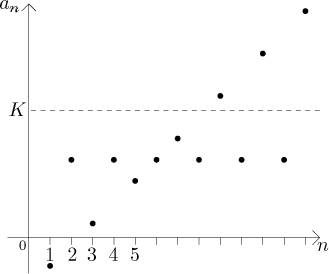This sequence is not bounded from above, so we would suspect that it tends to infinity, but every second term jumps down. Thus it seems that we can win some games, but when somebody chooses K as in the picture, we will be unable to cut away some beginning so that the tail of the sequence stays above K; there will always be points jumping below. This sequence does not tend to infinity. In fact, this sequence does not have any limit at all.

Although the sequences in the last two examples are divergent by the first definition of a limit (note that for both of them you cannot find a real number L that would work as a limit), we can see that having infinity (or negative infinity) as a limit still gives some useful information, you can form some idea about what is happening with the sequence.

Note that we had two different definitions of a limit, depending on what kind of a limit we looked at. We will try to put some system into it now:

Notation:
When a sequence has a limit, and the limit is a real number, then we say that the sequence is convergent. This limit is called a proper limit.
When a sequence has a limit, and the limit is infinity or negative infinity, then this limit is called an improper limit.
If a sequence has a limit, proper or improper, we say that the limit exists.
If a sequence has no limit at all, we say that the limit does not exist, this is often shortened as DNE.
Sequences with improper limit and sequences without limit are called divergent.

So there are two kinds of terminology and they overlap somewhat. The existence of a limit means that there is a definite tendency as n grows to infinity; the terms of the sequence may become almost equal to some number or they may have a definite tendency of going up (or down) without bound and staying there, but there is the tendency. Sequences whose limit DNE have no clear tendency; they may have two conflicting tendencies (see the latest picture above) or it may get even worse.

On the other hand, a convergent sequence has the property that for large values of n, its terms can be replaced with an appropriate number - its proper limit of course. Divergent sequences do not have this property. Since infinity is not really a number, sequences that tend to infinity or negative infinity must be classified as divergent, although they do have a limit (improper, that is). Actually, even if we took infinity as a number (which we will soon do in a sense), we still could not replace terms of such a sequence by infinity without making a great error; for instance, if we try to replace 2n by infinity, we will always make a big error, no matter how large n is; therefore this sequence is divergent, although it has a limit (infinity).

One last note: When using the "arrow notation", people very often skip the "as n→∞" part. After all, where else could n go? So we usually just write (1/2)n→0 or 2n→∞.

When we write anL, or say that "L is a limit" without closer specification, then L can be a real number or (plus or minus) infinity. Indeed, most properties are true for both proper and improper limits, so it makes sense to use a common notation. However, note that there is a difference in how we read it. We can say "an converges to L" only if we know that L is proper, that is, a real number. In general we say that "an tends to L", often people say informally "an goes to L".
On the other hand, when a sequence {an} does not have a limit, we would sometimes write "lim(an) DNE" (DNE as Does Not Exist). It is not exactly kosher, check with your prof whether it is O.K. to use this notation.

In this note we talked about an alternative way to draw sequences. We also introduce another way to look at a limit there, an alternative definition if you want. We suggest that you check it out.

We conclude this section with a theorem that is not exactly very useful but it shows that the notion of the limit is reasonable:

Theorem.
If a sequence has a limit, then this limit is unique.

In other words, it is not possible to have two different limits for one sequence.